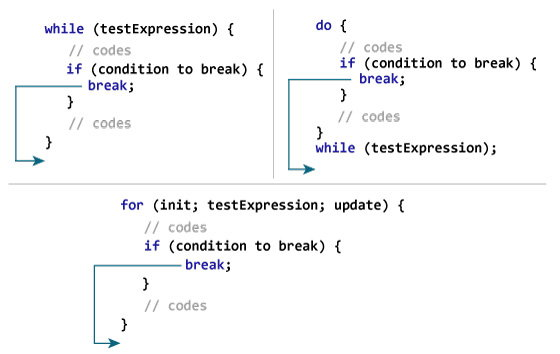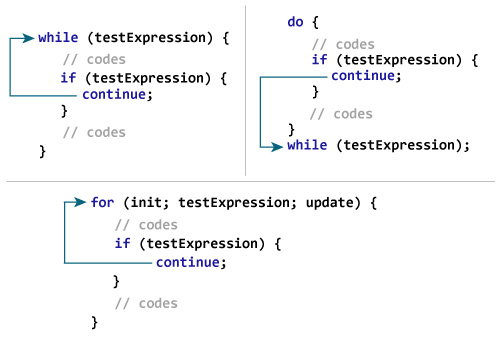C break and continue

# C break and continue

## C break

The break statement ends the loop immediately when it is encountered. Its syntax is:

``break;``

The break statement is almost always used with `if...else` statement inside the loop.

### How break statement works?### Example 1: break statement

``````// Program to calculate the sum of a maximum of 10 numbers
// If a negative number is entered, the loop terminates

# include <stdio.h>
int main()
{
int i;
double number, sum = 0.0;

for(i=1; i <= 10; ++i)
{
printf("Enter a n%d: ",i);
scanf("%lf",&number);

// If the user enters a negative number, the loop ends
if(number < 0.0)
{
break;
}

sum += number; // sum = sum + number;
}

printf("Sum = %.2lf",sum);

return 0;
}``````

Output

```Enter a n1: 2.4
Enter a n2: 4.5
Enter a n3: 3.4
Enter a n4: -3
Sum = 10.30```

This program calculates the sum of a maximum of 10 numbers. Why a maximum of 10 numbers? It's because if the user enters a negative number, the `break` statement is executed. This will end the `for` loop, and the sum is displayed.

In C, `break` is also used with the `switch` statement. This will be discussed in the next tutorial.

## C continue

The `continue` statement skips the current iteration of the loop and continues with the next iteration. Its syntax is:

``continue;``

The `continue` statement is almost always used with the `if...else` statement.

### How continue statement works?### Example 2: continue statement

``````// Program to calculate the sum of a maximum of 10 numbers
// Negative numbers are skipped from the calculation

# include <stdio.h>
int main()
{
int i;
double number, sum = 0.0;

for(i=1; i <= 10; ++i)
{
printf("Enter a n%d: ",i);
scanf("%lf",&number);

if(number < 0.0)
{
continue;
}

sum += number; // sum = sum + number;
}

printf("Sum = %.2lf",sum);

return 0;
}``````

Output

```Enter a n1: 1.1
Enter a n2: 2.2
Enter a n3: 5.5
Enter a n4: 4.4
Enter a n5: -3.4
Enter a n6: -45.5
Enter a n7: 34.5
Enter a n8: -4.2
Enter a n9: -1000
Enter a n10: 12
Sum = 59.70```

In this program, when the user enters a positive number, the sum is calculated using `sum += number;` statement.

When the user enters a negative number, the `continue` statement is executed and it skips the negative number from the calculation.